Learn to click the perfect selfie Learn to click the perfect selfie

# Selina Solutions for Class 9 Physics Chapter 9 - Current Electricity

Selina solutions for class 9 Chapter 9 Current Electricity provides complete information regarding sound waves and transmission of these waves through a medium. ICSE Class 9 students can refer to these solutions for this chapter and all the chapters as the solutions have been constructed with the information provided in the ICSE textbook in accordance to the C.IS.C.E.

Students get overwhelmed with the thought of examinations often and in a hurry to tick all the checkboxes when it comes to exam preparation, tend to forget what they had studied. Selina solutions serve as a single-window for all the academic requirements of class 9 students.

Solutions are designed in accordance with the latest syllabus prescribed by the board. Use of a simple language and easy to understand terminologies makes ICSE Class 9 Physics Selina solutions students’ favourite and hence perform exceedingly well at the examinations.

### Access Answers of Physics Selina class 9 Chapter 9 – Current Electricity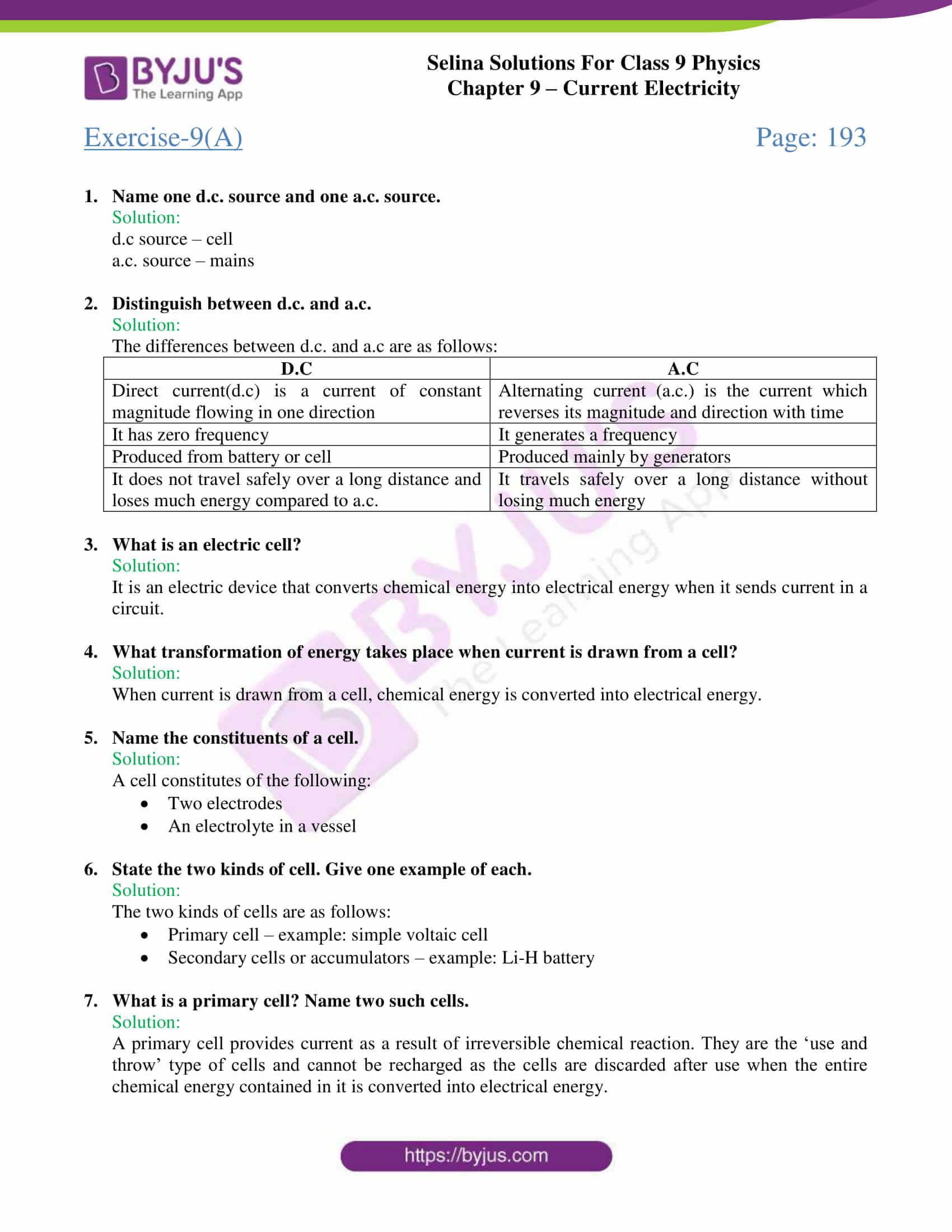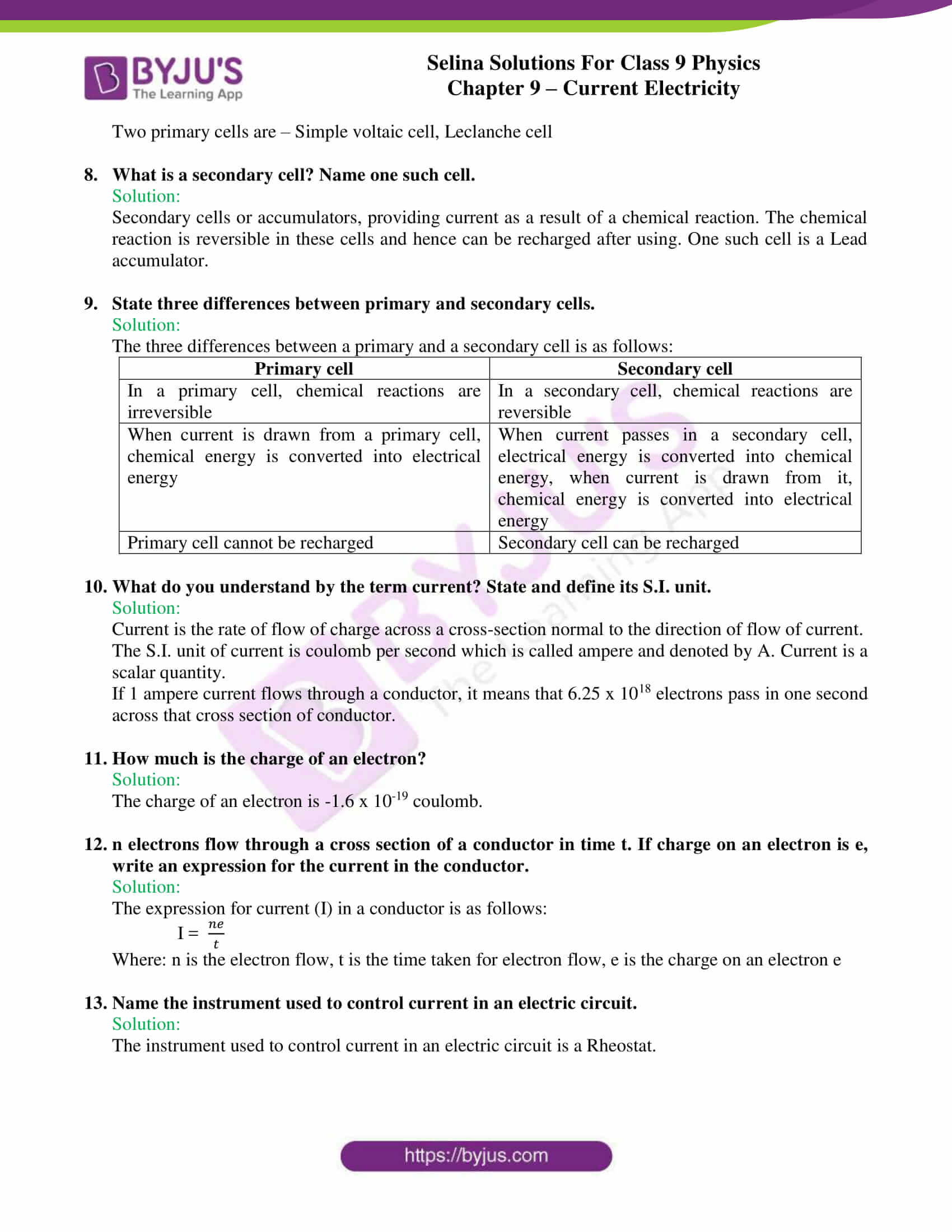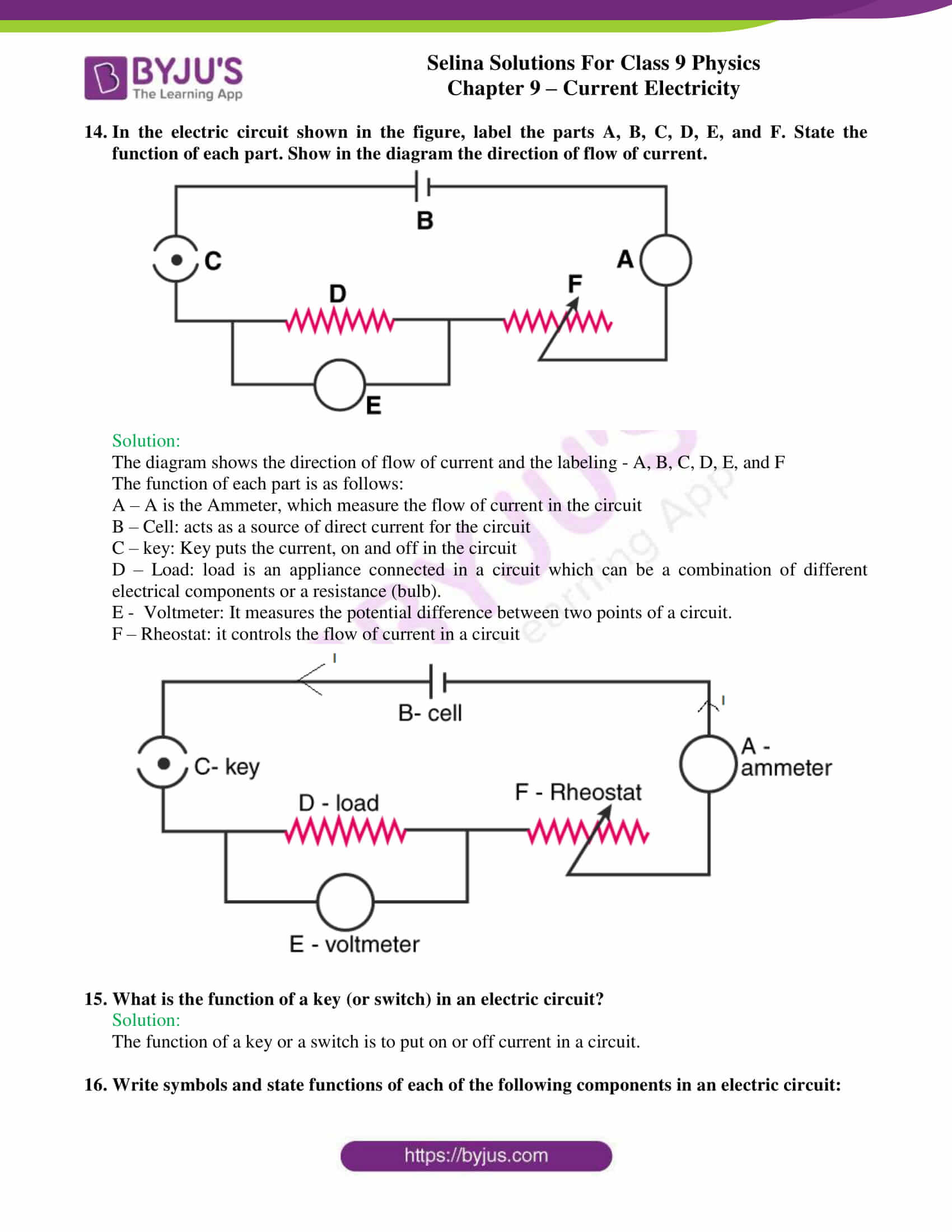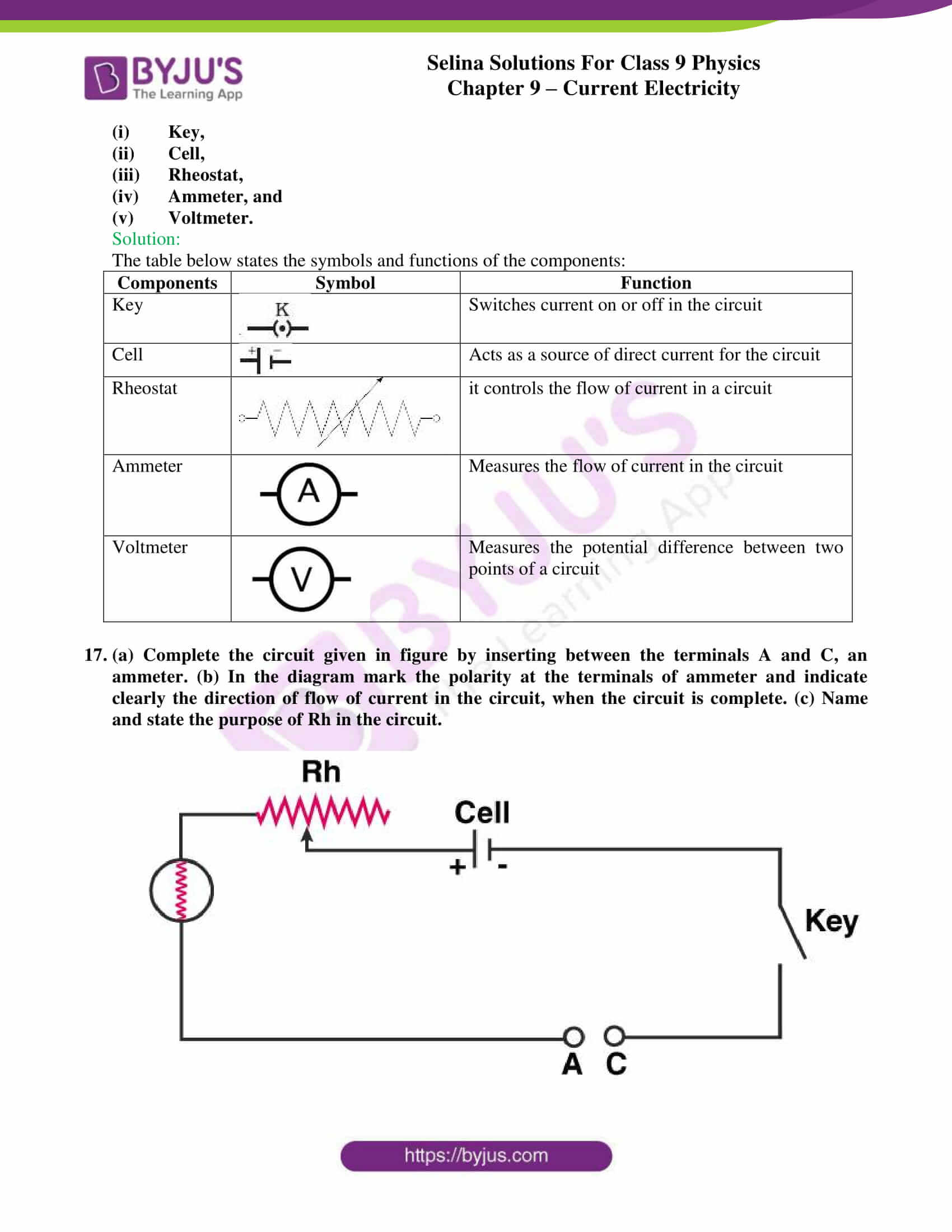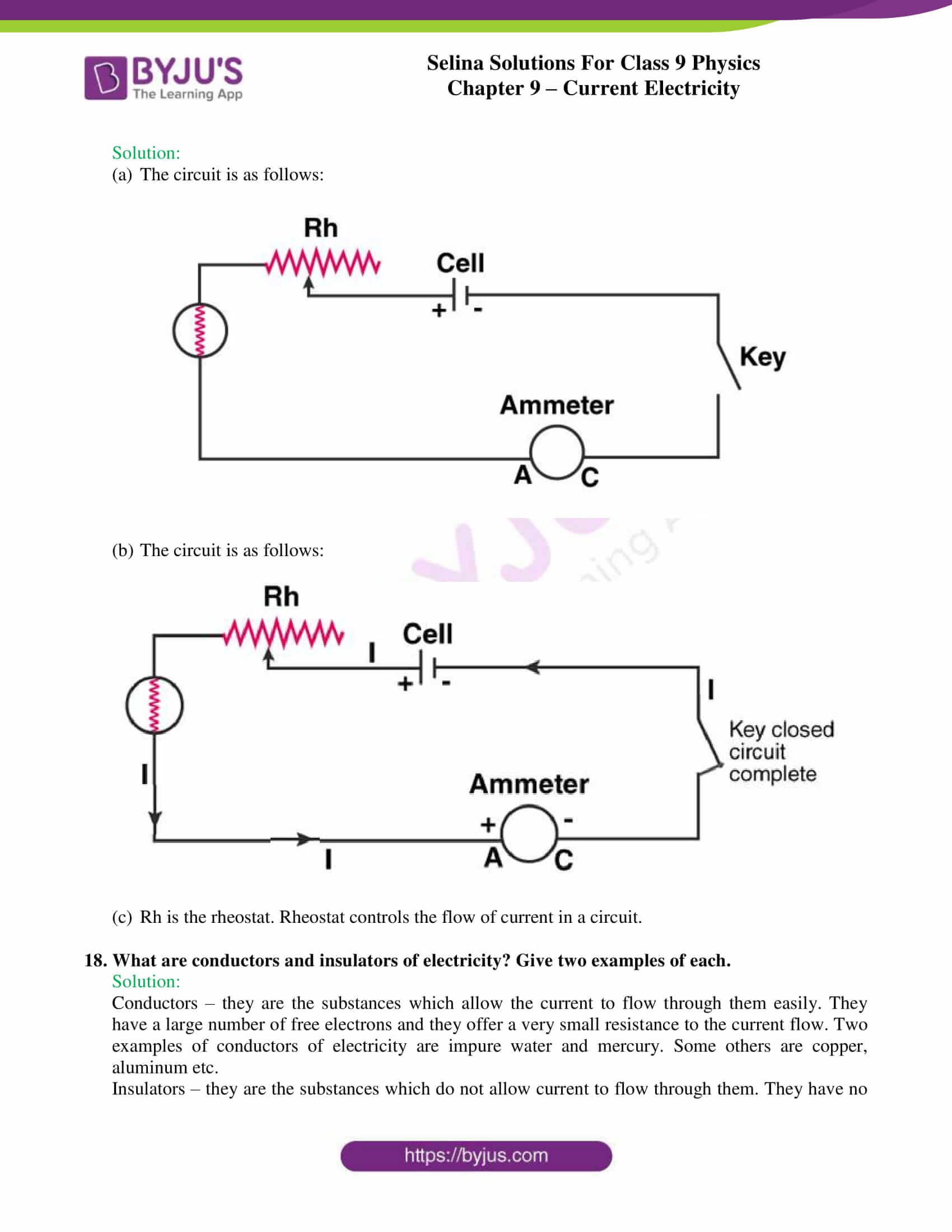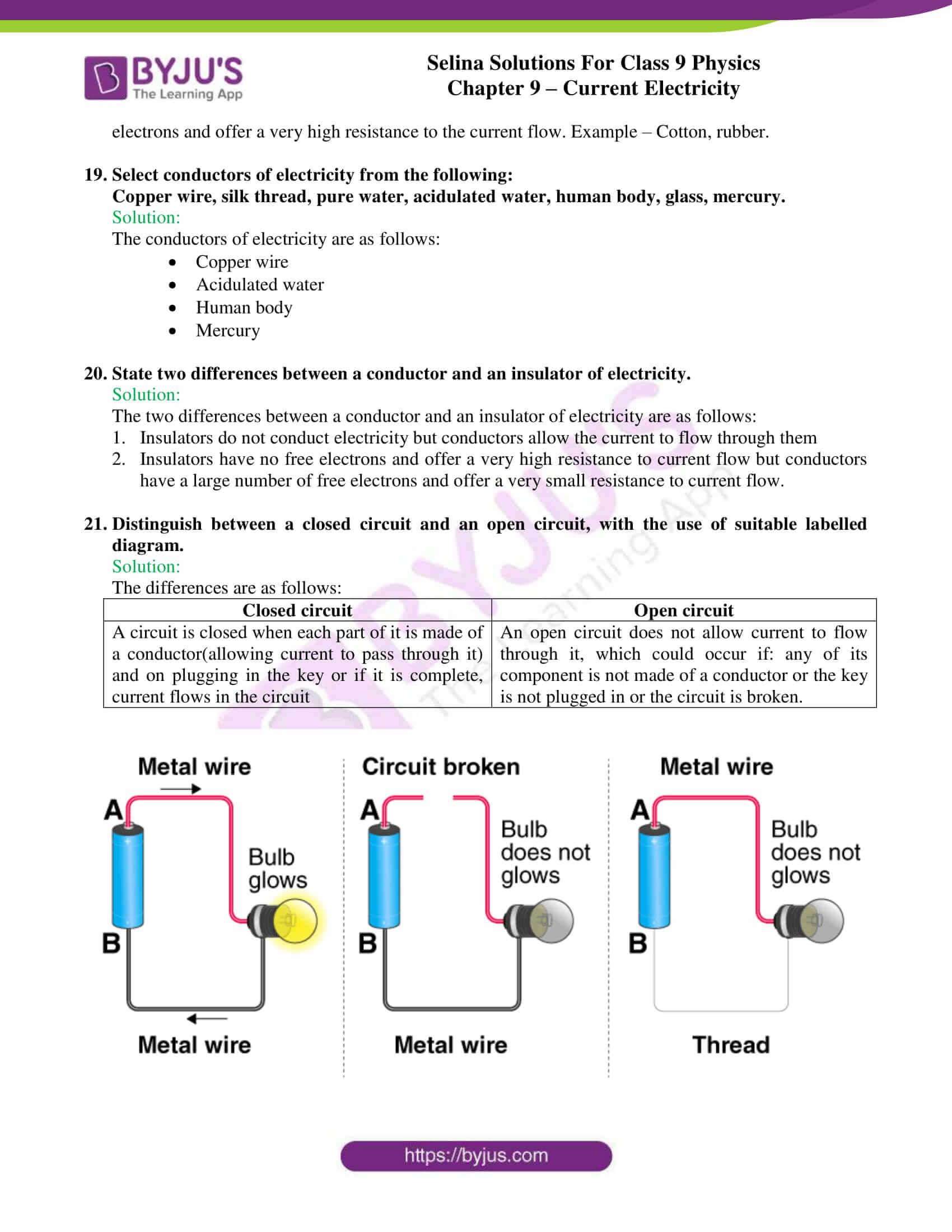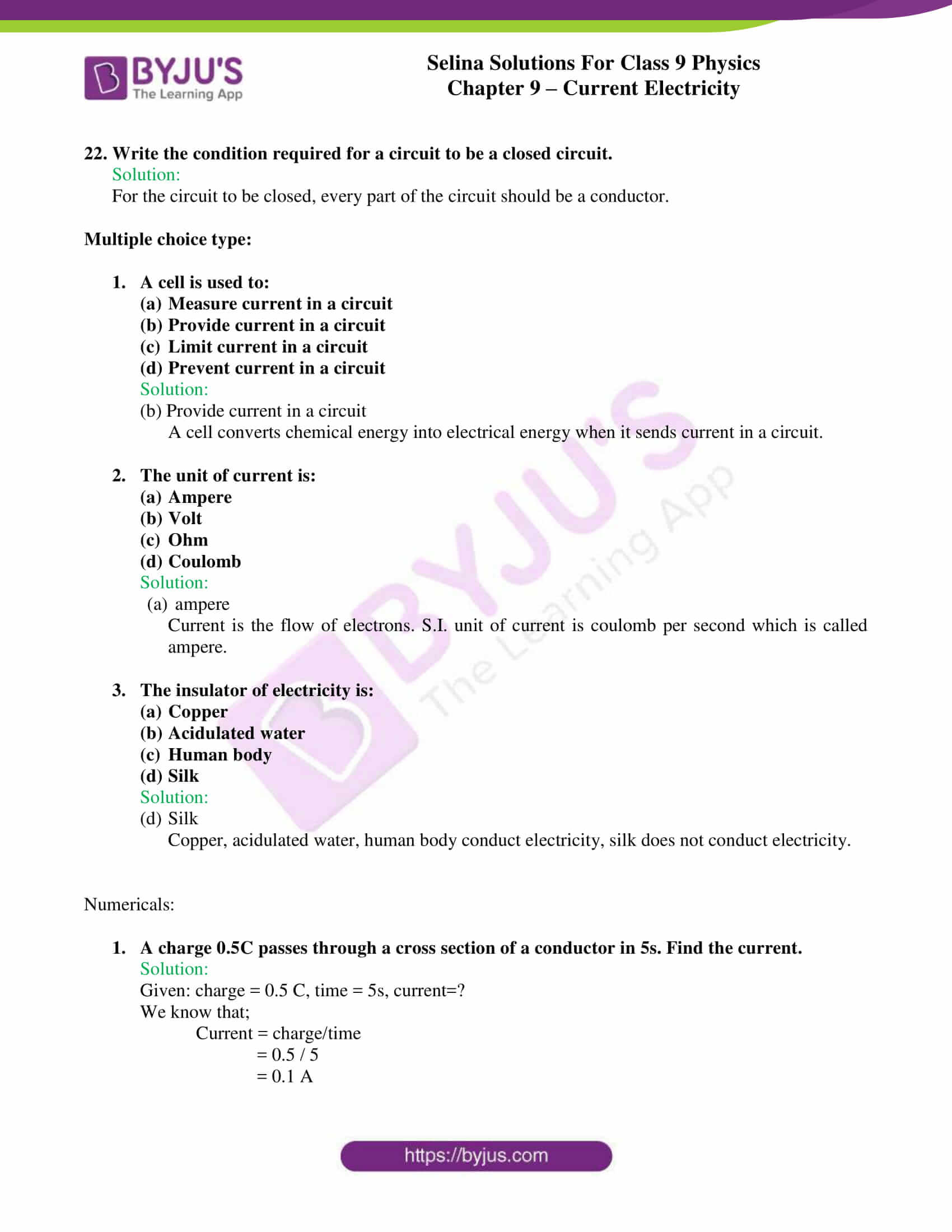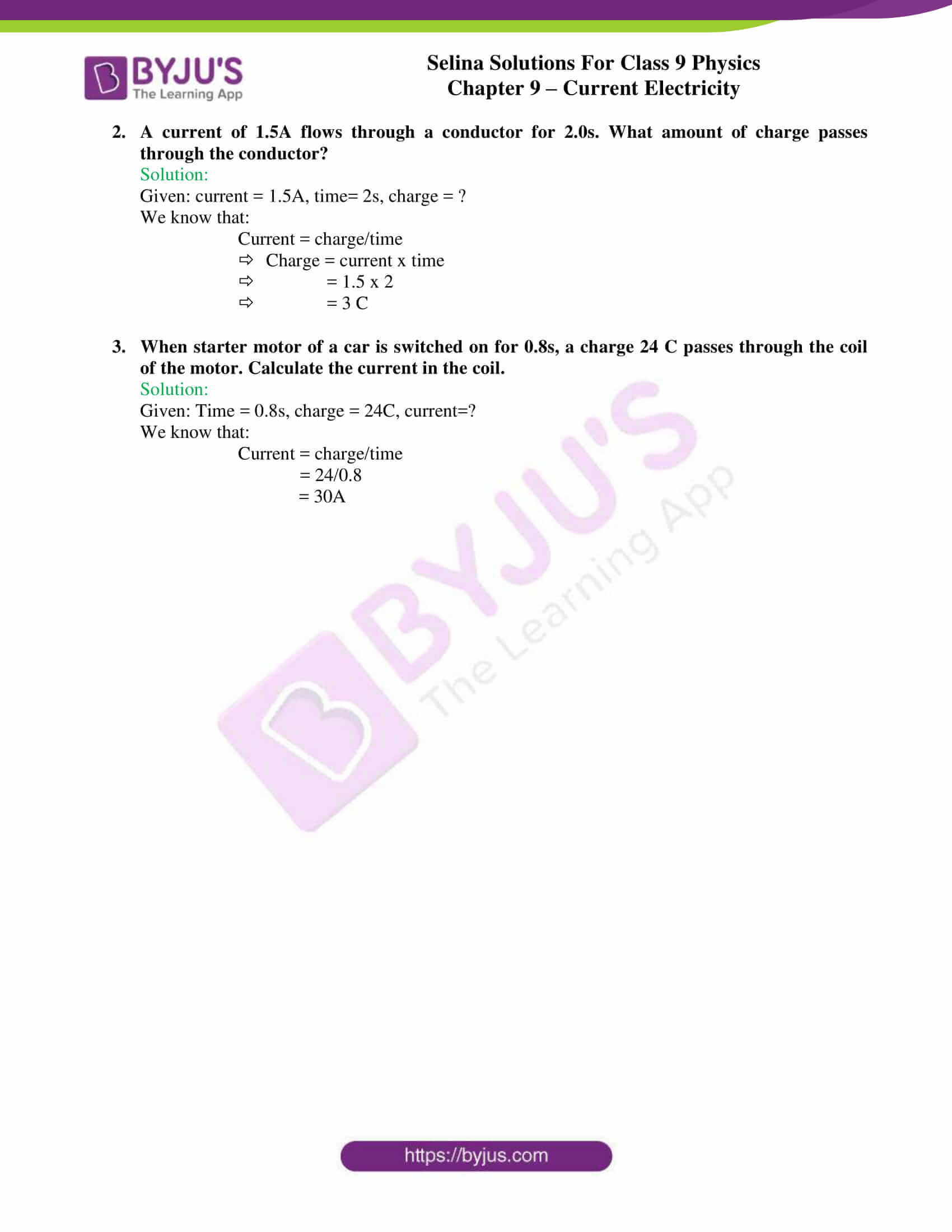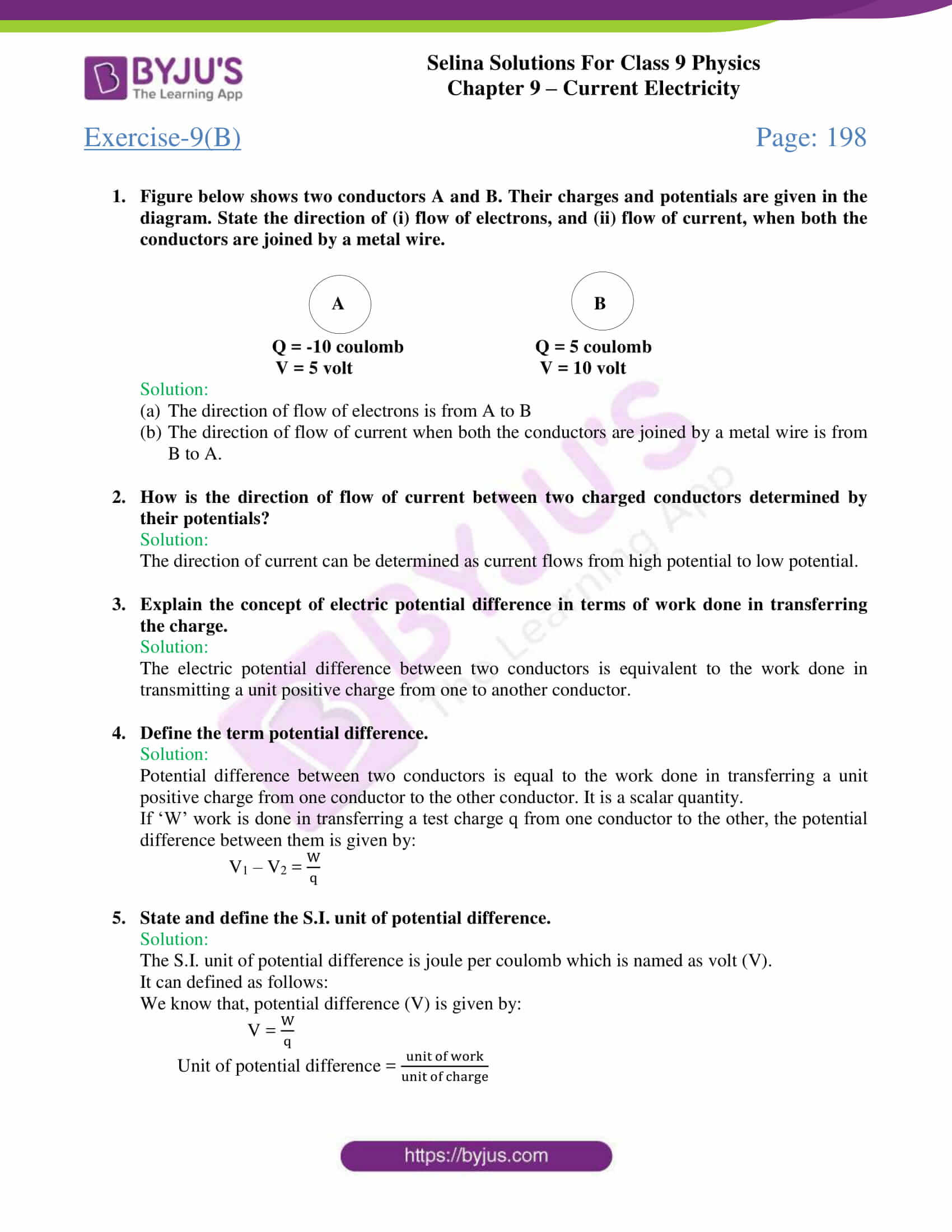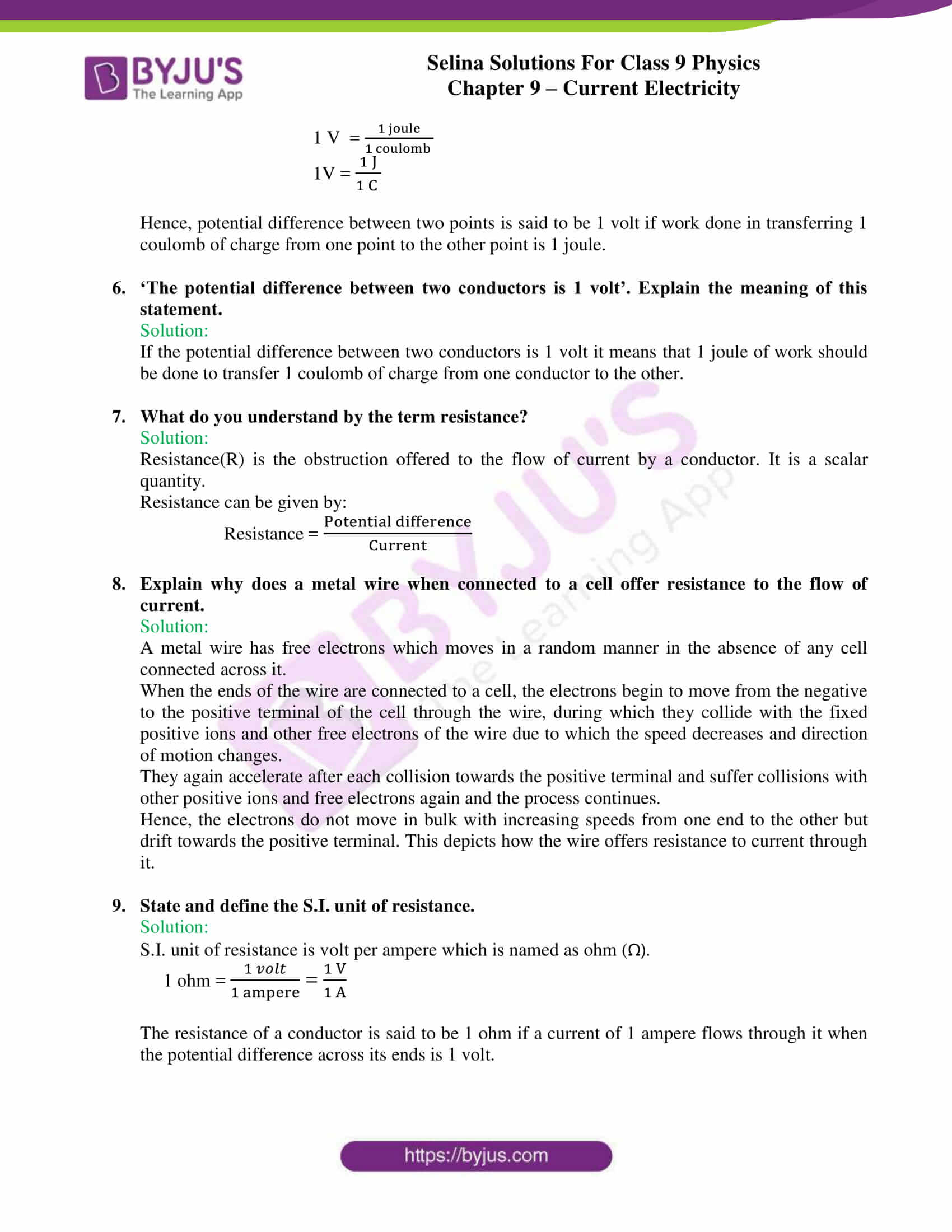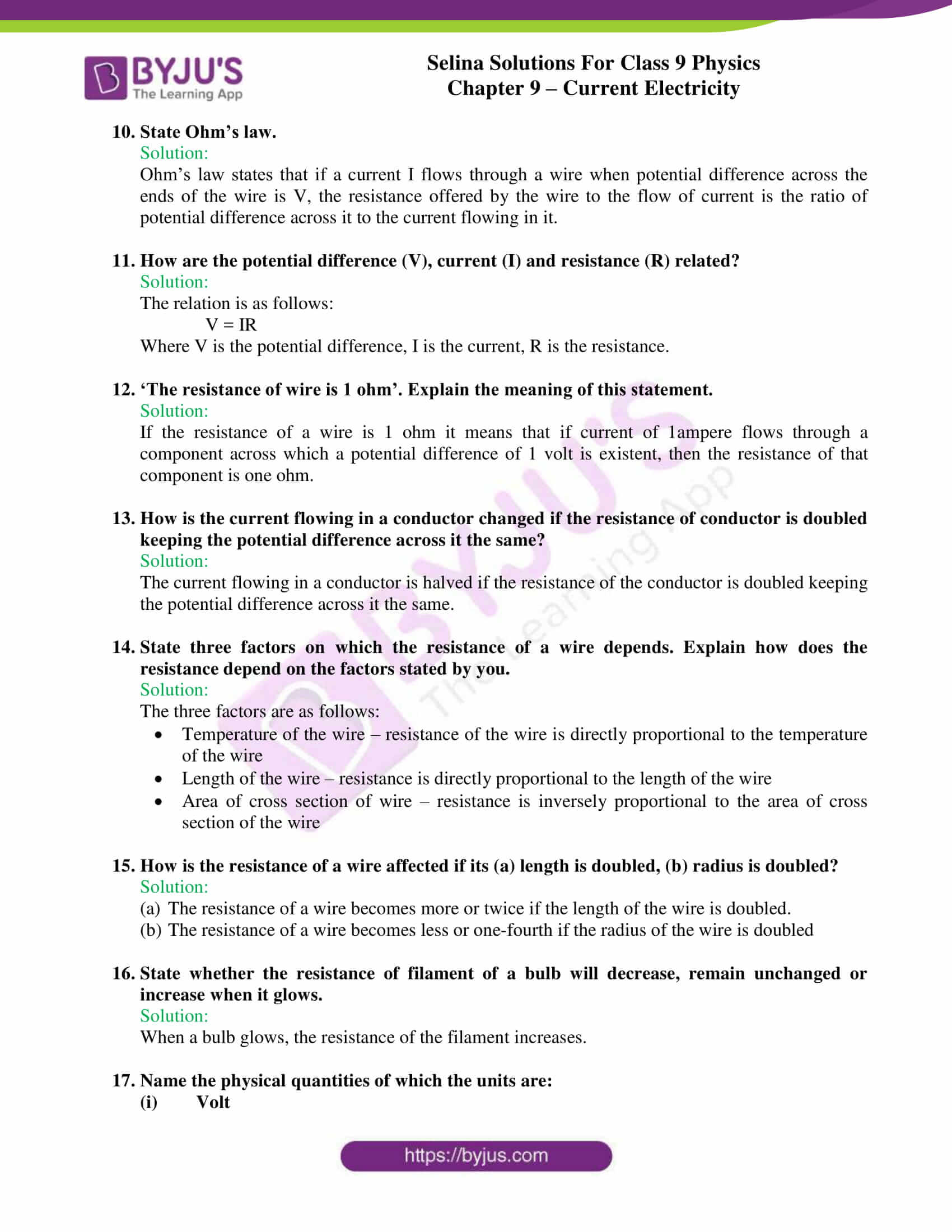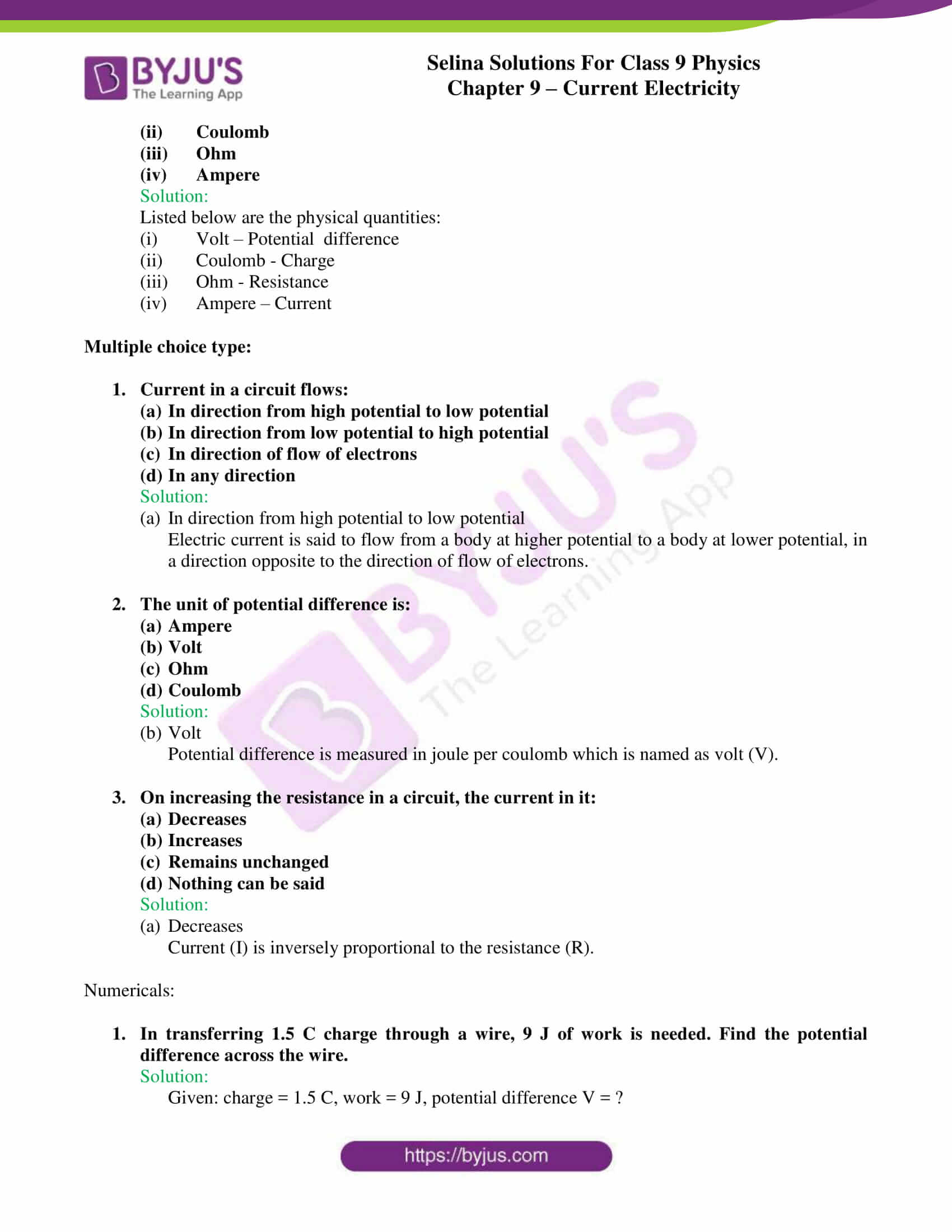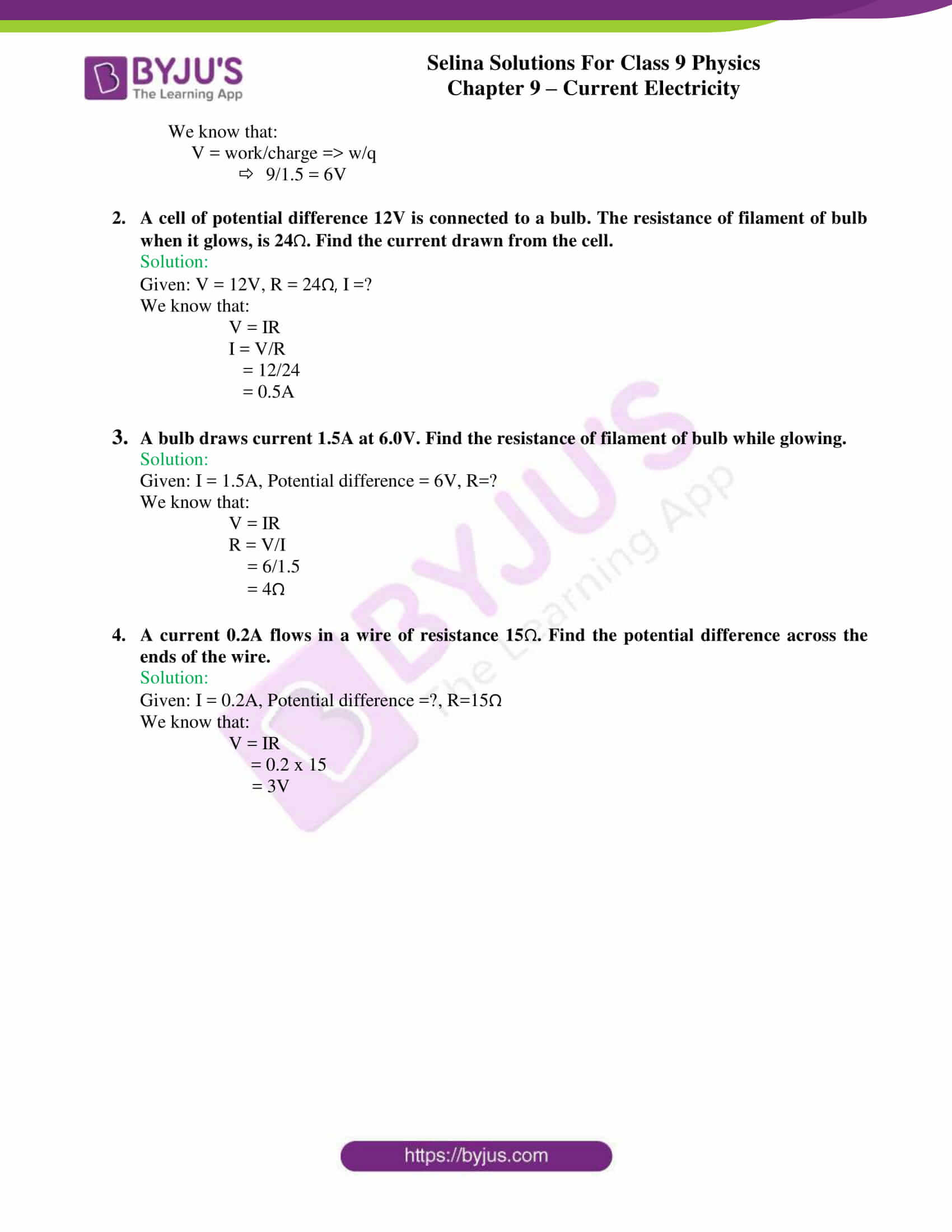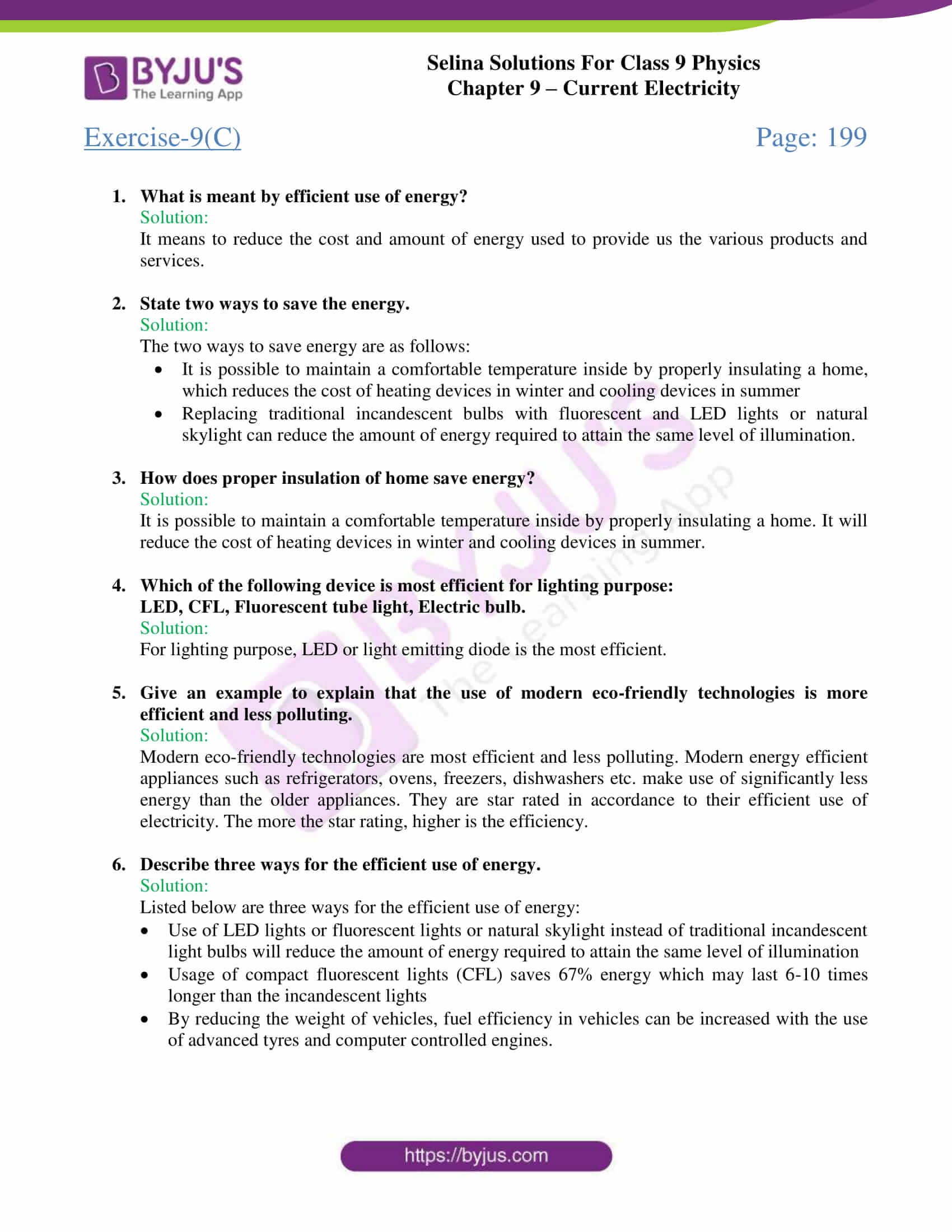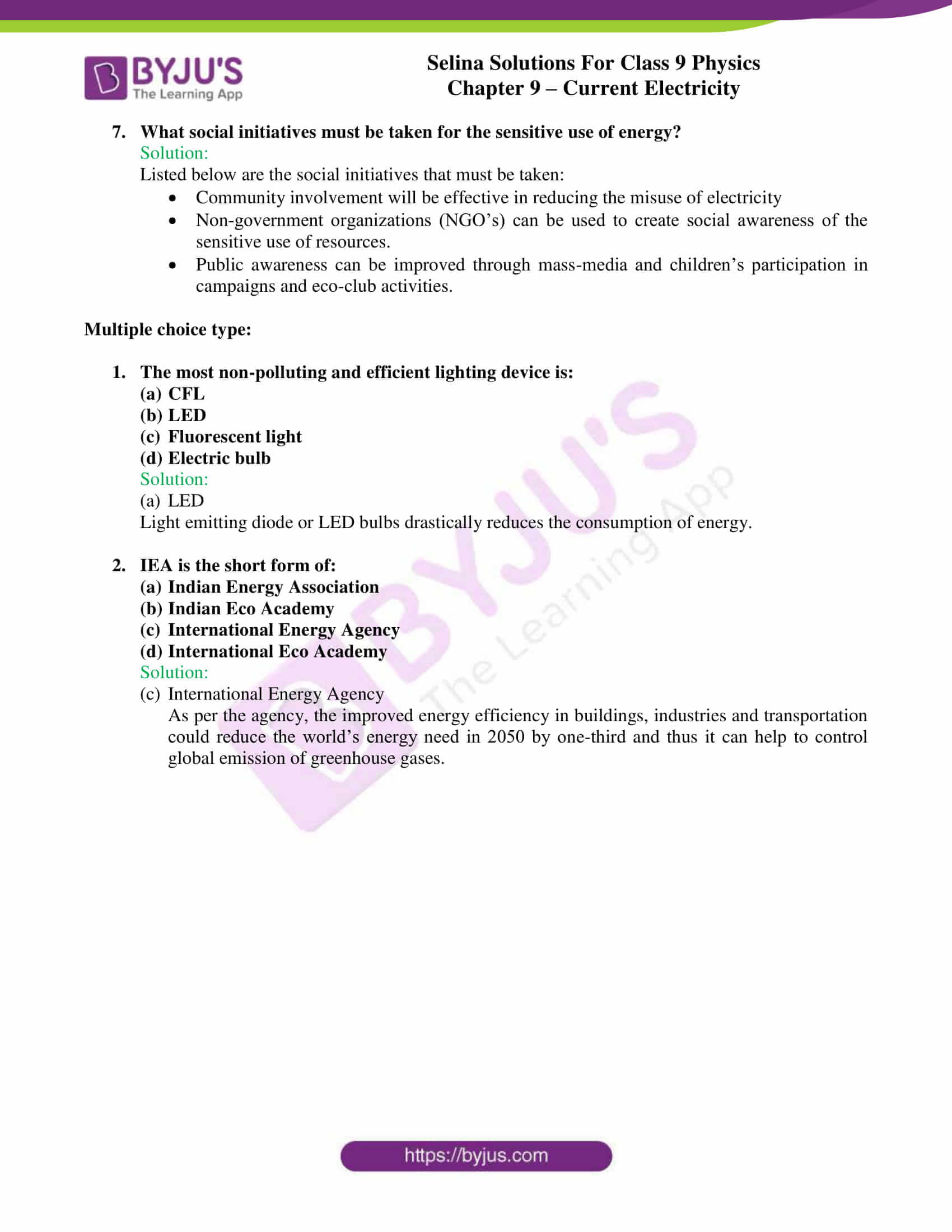## Selina Solutions for class 9 Physics Chapter 9 – Current Electricity

ICSE Class 9 Physics Chapter 9 Current electricity enlightens students with insights around the concepts in current electricity. Current is the rate of flow of charges. It discusses the two different types of sources of current (direct and alternating current) so that students understand their differences and their respective applications.

A current flows in a circuit. Current flows if the circuit is complete (Closed) not in an open circuit. The different symbols used in circuit diagrams are the source of current, key, rheostat, voltmeter, ammeter, load, galvanometer, connecting wires etc. It also briefs about components such as insulators and conductors etc. The next important topic discussed is the potential difference and resistance.

Finally, the efficient use of energy is discussed which briefs about the various ways that can be adapted to efficiently use energy resources that are available to us – use of CFL, LEDs, insulating homes properly, use of modern energy-efficient appliances are some of the initiatives that can be adapted.

List of subtopics covered in Chapter 9 – Current Electricity:

 Number Subtopic 9.1 Sources of direct current 9.2 Electric current 9.3 Symbols used in circuit diagrams 9.4 Simple electric circuit 9.5 Insulators and conductors 9.6 Closed and open circuits 9.7 Flow of electrons between the conductors 9.8 Direction of the electric current – conventional & electronic flow 9.9 Elementary idea about work done in transferring charge through a conducting wire; potential difference(V=W/Q) 9.10 Electrical resistance 9.11 Efficient use of energy 9.12 Social initiatives

List of Exercise

 Name of the exercise Number of questions Page number Exercise-9A 28 193 Exercise-9B 24 198 Exercise-9C 9 199

## Selina Solutions for class 9 Physics Chapter 9 – Current Electricity

Chapter 9 – Current electricity deals with everything about electricity. Current is the rate of flow of charges across a cross-section normal to the direction of flow of current. The topics explained in this chapter further are the use of a simple electric current with the help of electric cell and a bulb to introduce the idea of current, potential difference, insulators and conductors, closed an open circuits, direction of current etc.

Cells are of two kinds – primary cells and secondary cells. The chapter also discusses the differences between a primary cell and a secondary cell. Current is quantified in ampere. Students are presented with the different symbols used in circuit diagrams for the various electrical components such as the source of current, key, rheostat, ammeter, voltmeter, galvanometer, connecting wires etc.

Towards the end of each activity, students are provided with questions, example problems are numerical problems to assess their knowledge about electric current.

### Key Features of Selina Solutions for class 9 Physics 9 – Current Electricity

• Selina solutions are readily accessible and free
• Use of easy terms and expressions
• Numericals solved accurately
• Tables have been used wherever necessary
• Solutions crafted by subject matter experts after extensive research
• Solutions framed from information provided in the textbook

## Frequently Asked Questions on Current Electricity

### What is an electric cell?

It is an electric device that converts chemical energy into electrical energy when it sends current in a circuit.

### Name the constituents of a cell?

A cell constitutes of the following:

• Two electrodes
• An electrolyte in a vessel

### What is a secondary cell?

Secondary cells or accumulators, providing current as a result of a chemical reaction. The chemical reaction is reversible in these cells and hence can be recharged after use. One such cell is a Lead accumulator.

### Define the term potential difference?

Potential difference between two conductors is equal to the work done in transferring a unit positive charge from one conductor to the other conductor. It is a scalar quantity.

### State Ohm’s law?

Ohm’s law states that if a current I flows through a wire when the potential difference across the ends of the wire is V, the resistance offered by the wire to the flow of current is the ratio of potential difference across it to the current flowing in it.

### What is meant by efficient use of energy?

It means to reduce the cost and amount of energy used to provide us with the various products and services.